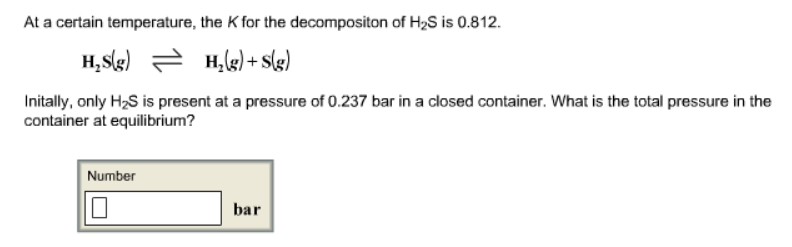# Problem: At a certain temperature, the K for the decomposition of H2S is 0.812. H2S (g) ⇌ H2 (g) + S (g) Initally, only H2S is present at a pressure of 0.237 bar in a closed container. What is the total pressure in the container at equilibrium?

###### FREE Expert Solution###### Problem Details

At a certain temperature, the K for the decomposition of H2S is 0.812.

H2S (g) ⇌ H2 (g) + S (g)

Initally, only H2S is present at a pressure of 0.237 bar in a closed container. What is the total pressure in the container at equilibrium?